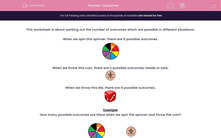# Outcomes

In this worksheet, students work out how many different outcomes are possible in each situation.Key stage:  KS 2

Curriculum topic:   Maths and Numerical Reasoning

Curriculum subtopic:   Probability

Difficulty level:#### Worksheet Overview

This worksheet is about working out the number of outcomes which are possible in different situations.

When we spin this spinner, there are 8 possible outcomes.When we throw this coin, there are 2 possible outcomes, heads or tails.When we throw this die, there are 6 possible outcomes.Example

How many possible outcomes are there when we spin the spinner and throw the coin?For each colour, we can get heads or we can get tails.

The number of outcomes = 8 x 2 = 16

### What is EdPlace?

We're your National Curriculum aligned online education content provider helping each child succeed in English, maths and science from year 1 to GCSE. With an EdPlace account you’ll be able to track and measure progress, helping each child achieve their best. We build confidence and attainment by personalising each child’s learning at a level that suits them.

Get started